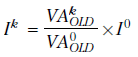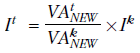6429.0 - Producer and International Trade Price Indexes: Concepts, Sources and Methods, 2014
Latest ISSUE Released at 11:30 AM (CANBERRA TIME) 20/08/2014
Page tools:Print AllRSSSearch this Product

CHAPTER 12 LINKING AND RE–REFERENCING

INTRODUCTION

12.1 The Australian Producer Price Indexes (PPIs) and International Trade Price Indexes (ITPIs) are periodically reviewed (see Chapter 11) to account for substitution in the marketplace, the evolution of pricing mechanisms practiced by different businesses and the emergence of new products. This chapter describes the reference periods used in the PPIs and ITPIs and the chain linking process.

REFERENCE PERIODS

12.2 The following reference periods are discussed in this chapter:

• Weight reference period is the period covered by the expenditure statistics used to calculate the weights
• Price reference period is the period for which prices are used as denominators in the index calculation
• Index reference period is the period for which the index is set to 100.0.

RE-REFERENCING

12.3 The Australian Bureau of Statistics (ABS) changes the index reference period (a process known as re-referencing) of the PPIs and ITPIs from time to time, but not frequently. This is because frequently changing the index reference period is inconvenient for users, particularly those who use the PPIs and ITPIs for contract escalation. Re-referencing results in the loss of precision for historic data, especially for long series. The current PPI and ITPI index reference period is 2011-12 = 100.0.

12.4 The conversion of an index series from one index reference period to another involves calculating a conversion factor using the ratio between the two series of index numbers. For example, converting the Stage of Production Intermediate demand: total from an index reference period of 1998-99 = 100.0 to 2011-12 = 100.0 (see Table 12.1). The index number for the 2011-12 Stage of Production Intermediate demand: total using an index reference period of 1998-99 is (150.2 + 150.7 + 151.1 + 152.2)/4 = 151.1. (rounded to one decimal place). Using the conversion factor is 0.6620 (100.0/151.1), the March quarter 2011 index number based on an index reference period of 2011-12 = 100.0 is 97.3 (147.0×0.6620).

 Table 12.1 Converting index reference periods, Stage of Production Intermediate demand: totalIndex reference period(a) Period 1998-99=100.0 2011-12=100.0 Mar qtr 2011 147 97 Jun qtr 2011 150 99 Sep qtr 2011 150 99 Dec qtr 2011 151 100 Mar qtr 2012 151 100 Jun qtr 2012 152 101 Financial year 2011-12 151 100 Sep qtr 2012 153 101 Dec qtr 2012 153 102 Mar qtr 2013 153 102 Jun qtr 2013 154 102 Sep qtr 2013 156 103 Dec qtr 2013 157 104 (a) Conversion factor: 1998-99 index reference period to 2011-12 index reference period = 100.0/151.1 = 0.6620.

12.5 Similar procedures are used to convert the 2011-12 index reference period to a 1998-99 index reference period. For example, the December quarter 2013 index for the Stage of Production Intermediate demand: total was 103.6 which, when multiplied by the conversion factor of 1.511 (151.1/100.0), gives an index number of 156.5 on the index reference period of 1998-99 = 100.0. It should be noted that a different conversion factor will apply for each index - that is, the factor for the Stage of Production Intermediate demand: total will differ from the factor for Stage of Production Preliminary demand: total and Stage of Production Final demand: total.

12.6 Re-referencing should not be confused with reweighting. Re-referencing does not change the relative movements between periods. However reweighting involves introducing new weights and recalculating the aggregate index for each period which will affect the relative movements between periods.

Rounding and loss of precision

12.7 Loss of precision in long-term historic series arises as a result of this process because published price index data are rounded and stored to one decimal place. This practice of rounding and storing historical index series to one decimal place is currently under review by the ABS.

12.8 Published percentage changes to index numbers are calculated from the rounded index numbers. A consequence of re-referencing price indexes can be that period-to-period percentage changes may differ to those previously published due to rounding of the re-referenced values. These differences do not constitute a revision.

INCORPORATING A REVIEW INTO AN INDEX

12.9 To incorporate new weighting data into an existing price index, three steps must be undertaken: a link period must be chosen, value data must be price updated to the link period, and the newly weighted index chained onto the existing price index.

The link period

12.10 The link period for the PPIs and ITPIs is a quarter in which the index is calculated on both the old and new weighting structures. The link period is based on availability and timing of data, internal resource constraints, economic behaviour and should not coincide with changes to tax legislation or other significant regulatory amendments.

12.11 The June quarter is frequently chosen as the link period for these reasons as this choice allows detailed comparisons of complete financial years without having to account for the link. The ITPIs are reweighted each year to align to trade data and chain linked every year through the June quarter.

Price updating value data

12.12 Weights are price updated to account for price changes between the weight reference period and the link period (see Chapter 6). Weighting data usually comes from an annual data source, and in some cases can span more than one year. The link period, however, is always chosen to be a single quarter, and is always a period subsequent to the weight reference period. Although only observed in aggregate, the value data can be considered as being composed of the product of prices and quantities from the weight reference period. For inclusion in the index, these data will be expressed in terms of quantities from the weight reference period and prices from the link period.

12.13 Price updating value data is achieved by multiplying the weighting data by the proportional price change between the weight period and the link period. This updating occurs at the elementary aggregate level, with the resulting upper level value aggregates determined by aggregating the price updated components along the index structure.

12.14 The proportional price change between the weight period and the link period is determined by the ratio of the price indexes for the two periods; here the price indexes are on the existing index structure. In the usual case where the weighting reference period is longer than a quarter, the price index for the weight period is determined as the average of the quarterly price indexes that the weighting period spans. The ratio is the link period index divided by the averaged weight period index.

12.15 The resulting link period value aggregate is then expressed in terms of prices from the link period and quantities from the weight reference period.

Chain linking through a link period

12.16 Chain linking can best be illustrated by means of an example. In this example we will consider a price index at period k, with the index constructed from weights introduced in the reference period 0. Using the terminology from Chapter 10, we would express the price index at period k asIf we choose period k as the link period, any future period price indexes will make comparisons back to the link period, and be scaled by the link period index Ik. Any such comparisons will use the same price index Ik but use a value aggregate calculated on the new weighting basis. If we consider t, some period after k, a price index measuring the average price change from period 0 to period t is given byNew components

12.17 The introduction of new elementary aggregates (or any other component) into a price index during a sample review follows the same general principles, however any new elementary aggregate will not have a price index for the link period under the old structure.

Example of chain linking

12.18 The use of fixed weights (as in a Lowe formula) over a long period of time is not considered a sound practice. For example, weights in a producer price index have to be changed to reflect changing production patterns. Production patterns change in response to longer term price movements, changes in preferences, and the introduction or displacement of products.

12.19 The PPIs and ITPIs use a Lowe index. There are two options for updating weights. Option one, known as the direct method, involves holding the weights constant over as long a period as seems reasonable, starting a new index each time the weights are changed. This means that a longer term series is not available. Option two is to update the weights more frequently and chain link the series together to form a long-term series. The latter is the method used for ABS price indexes.

12.20 The behaviour under chain linking of the Lowe index formula is explored in Table 12.2.

 TABLE 12.2 A CLOSER LOOK AT LINKING Item Period 0 Period 1 Period 2 Period 3 Period 4 PRICE (\$) Electricity 10 12 15 10 15 Gas 12 13 14 12 10 Water 15 17 18 15 12 QUANTITY Electricity 20 17 12 20 10 Gas 15 15 16 15 20 Water 10 12 8 10 15 INDEX NUMBER Index FormulaLoweperiod 0 to 1 100.0 114.2period 1 to 2100.0 112.9period 2 to 3100.0 78.8period 3 to 4100.0 107.5chain 100.0 114.2 128.9 101.6 109.2direct 100.0 114.2 130.2 100.0 107.5

12.21 In period 3, prices and quantities are returned to their index reference period values and in period 4 the index reference period prices and quantities are shuffled between items. The period 3 situation is sometimes described as time reversal and the period 4 situation as price bouncing.

12.22 The index number under direct estimation returns to 100.0 when prices and quantities of each item return to their index reference period levels; however, the chained index numbers do not.

12.23 This situation poses a quandary for prices statisticians when using a fixed weighted index. There are obvious attractions in frequent chaining; however, chaining in a fixed weighted index may lead to biased estimates. This can occur if there is seasonality or cycles in the price, and chaining coincides with the top or bottom of each cycle. For this reason it is generally accepted that indexes should not be chained at intervals less than annual.